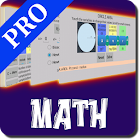Interactive Math PRO

All Android applications categories

All Android games categories# Interactive Math PRO

by: 1 10

10 Users
rating

## Screenshots

Description

"Interactive Math PRO" is ideal for students and graduates, you will find a variety of useful formulas and tools for your daily calculus, you can enter a value by touch and solve any variable of the equation, the interactive feature of the formulas make it easy to avoid typing or human errors.
The interface is made in a way that you can see above any variable it's value.

TOPICS OF THIS MATH SOLVER:
"GEOMETRY-RECTANGLE PERIMETER",
"GEOMETRY-RECTANGLE AREA",
"GEOMETRY-TRIANGLE PERIMETER",
"GEOMETRY-TRIANGLE AREA",
"GEOMETRY-PARALLELOGRAM PERIMETER",
"GEOMETRY-PARALLELOGRAM AREA",
"GEOMETRY-TRAPEZOID PERIMETER",
"GEOMETRY-TRAPEZOID AREA",
"GEOMETRY-CIRCLE PERIMETER",
"GEOMETRY-CIRCLE AREA",
"GEOMETRY-CIRCLE SECTOR LENGTH",
"GEOMETRY-CIRCLE SECTOR AREA",
"GEOMETRY-ELLIPSE AREA",
"GEOMETRY-RECTANGULAR PARALLELEPIPED VOLUME",
"GEOMETRY-SPHERE SURFACE",
"GEOMETRY-SPHERE VOLUME",
"GEOMETRY-CYLINDER LATERAL SURFACE",
"GEOMETRY-CYLINDER VOLUME",
"GEOMETRY-CONE VOLUME",
"GEOMETRY-PYRAMID VOLUME",
"GEOMETRY-ELLIPSOID VOLUME",
"GEOMETRY-DISTANCE BETWEEN 2 POINTS",
"GEOMETRY-SLOPE BETWEEN 2 POINTS",
"GEOMETRY-RECTANGULAR-POLAR COORDINATES CONVERSION",
"SQUARE MATRIX OPERATIONS",
"SYSTEM EQUATIONS RESOLUTION",
"ANY MATRIX OPERATIONS",
"PROBABILITY-BINOMIAL DISTRIBUTION",
"2nd DEGREE EQUATION RESOLUTION",
"3rd DEGREE EQUATION RESOLUTION",
"EQUATION RESOLUTION (BISECTION & NEWTON)",
"PROBABILITY-HYPERGEOMETRIC DISTRIBUTION",
"INTEGRATION (SINGLE VARIABLE)",
"PROBABILITY-POISSON DISTRIBUTION",
"PROBABILITY-STANDARD NORMAL DISTRIBUTION",
"PROBABILITY-t DISTRIBUTION",
"PROBABILITY-X2 DISTRIBUTION",
"STATISTICS ONE VARIABLE",
"STATISTICS TWO VARIABLES",
"GEOMETRY-PYTHAGOREAN THEOREM",